# Noether's theorem

Noether's theorem is a mathematical result which relates continuous symmetries and conserved quantities in mechanical systems. For instance, physical laws are independent of location; this symmetry implies that the momentum is constant. Noether's theorem was published by Emmy Noether in 1918.

##  Informal description

There are several formulations of Noether's theorem, but the basic principle is always the same: continuous symmetries correspond to conserved quantities in variational systems. We see that three concepts have to be explained before we can understand the result. What are continuous symmetries, conserved quantities and variational systems?

Of these, conserved quantity is the easiest to understand. They are numbers associated to the system under study which do not change in time, even though the components of the system may move around. In our example, the conserved quantity is momentum. The momentum of an object is the product of its mass and its velocity, while the momentum of a system is the sum of the momenta of all objects that comprise the system.

Let us now have a look at continuous symmetry. In science, a symmetry of a system means that it behaves the same under some transformation. For instance, it does not matter if a marble rolls from left to right or from right to left on a flat table; that is why the world still looks natural if you look in a mirror. This is an example of a discrete symmetry, because there are only two possibilities: you exchange left or right, or you do not. An example of a continuous symmetry is that the marble behaves the same, no matter what position it starts from. This is called translational invariance, and it covers infinitely many transformations: shifting the system one millimeter to the left, or an inch to the right, and so on. Noether's law only works for continuous symmetries.

The final concept is that of a variational system. This is the most abstract concept, but the important point is that most mechanical systems fall within the class of variational systems. These systems satisfy a principle of least action, which means that the system evolves in such a way that its action is minimal (or, more precisely, stationary). For instance, Fermat's principle states that light takes that path which it can transverse in the least time.

Roughly, Noether's theorem says that if a variational system allows a continuous symmetry, then the corresponding quantity is conserved. A precise explanation of Noether's theorem requires the precise definitions of the key concepts of conserved quantity, continuous symmetry, and variational system.

##  Conservation of momentum in Newtonian mechanics

Let us first look at a simple example before discussing the Noether's theorem in its abstract form.

Consider a system consisting of two point masses. Denote their masses by m1 and m2 and their positions by x1 and x2. Newton's second law states that the acceleration of the masses is given by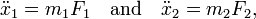$\ddot{x}_1 = m_1 F_1 \quad\mbox{and}\quad \ddot{x}_2 = m_2 F_2,$

where F1 is the force exerted on the first particle, and F2 the force on the second particle.

We assume that the force is conservative. This means that there exists a potential energy V(x1,x2) such that the force is (minus) the gradient of the potential energy: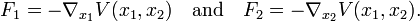$F_1 = -\nabla_{x_1} V(x_1,x_2) \quad\mbox{and}\quad F_2 = -\nabla_{x_2} V(x_1,x_2).$

This assumption ensures that our system has a variational structure.

Now, suppose that the potential energy is translationally invariant. This means that the potential energy does not change if we displace all the particles over the same distance v. In symbols, for any vector v, we have$V(x_1,x_2) = V(x_1+v,x_2+v). \,$

For instance, the gravitational potential is of this form. Since the force is the gradient of the potential energy, translation invariance implies that the forces between the particles remain the same if the system is moved as a whole.

Noether's theorem says that under these assumptions, the total momentum is conserved. The momentum of one particle is the product of its mass and its velocity, and the total momentum is the sum of all momenta: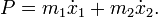$P = m_1 \dot{x}_1 + m_2 \dot{x}_2.$

This quantity remains constant.

In fact, that is not so hard to prove. The total momentum is constant if its time derivative is zero, so let's compute this derivative: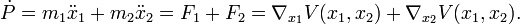$\dot{P} = m_1 \ddot{x}_1 + m_2 \ddot{x}_2 = F_1 + F_2 = \nabla_{x_1} V(x_1,x_2) + \nabla_{x_2} V(x_1,x_2).$

Here, we used the definition of the total momentum, Newton's second law, and the fact that the force arises from a potential. Now use the translation invariance to get:$\nabla_{x_1} V(x_1,x_2) + \nabla_{x_2} V(x_1,x_2) = \nabla_{x_1} V(x_1-x_2,0) + \nabla_{x_2} V(x_1-x_2,0).$

The first term is the gradient of V with respect to its first argument, and the second term is its negative. Hence, their sum is zero and the total momentum is conserved.

We conclude that if the force is conservative and the potential is translationally invariant, then the total momentum is conserved. The results (and its proof) carries over for systems with more than two particles.

##  Noether's theorem in Lagrangian mechanics

The central object in Lagrangian mechanics is the Lagrangian function$L(q,\dot{q})$, which is a function of the generalized coordinates qRn, the generalized velocities$\dot{q}$ and time t. The evolution of the coordinates is then given by the Euler-Lagrange equation:$\frac{\mathrm{d}}{\mathrm{d}t} \frac{\partial L}{\partial\dot{q}_i} = \frac{\partial L}{\partial q_i}.$

The example in the previous section can be brought in the Lagrangian framework by taking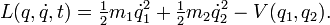$L(q,\dot{q},t) = \tfrac12 m_1 \dot{q}_1^2 + \tfrac12 m_2 \dot{q}_2^2 - V(q_1,q_2).$

A symmetry in this context is a one-parameter group of diffeomorphisms. This one-parameter group consists of diffeomorphisms φs: RnRn for every sRn, such that φ0 is the identity and φs+t = φs o φt. A Lagrangian system admits the one-parameter group hs as a symmetry if it does not change under the action of the diffeomorphisms: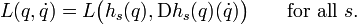$L(q,\dot{q}) = L \big( h_s(q), \mathrm{D}h_s(q)(\dot{q}) \big) \qquad\text{for all } s.$

Noether's theorem says that there is a first integral (that is, a preserved quantity) associated with every symmetry.

Noether's theorem in Lagrangian mechanics: If the system with Lagrangian L admits the symmetry hs, then the function I: Rn × RnR defined by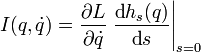$I(q,\dot{q}) = \frac{\partial L}{\partial\dot{q}} \left. \frac{\textrm{d}h_s(q)}{\textrm{d}s} \right|_{s=0}$

is a first integral.